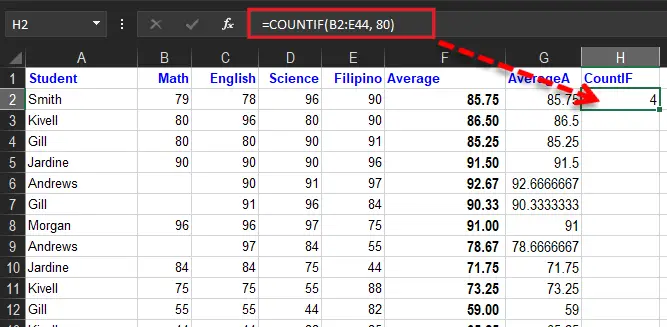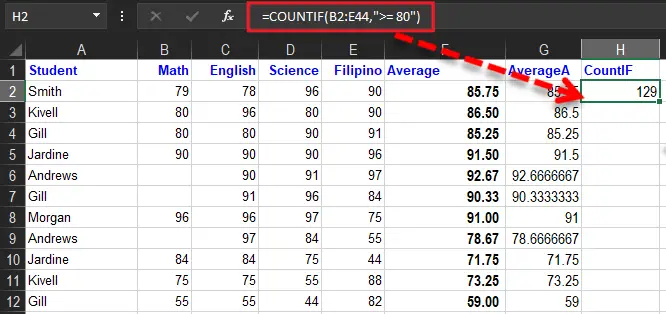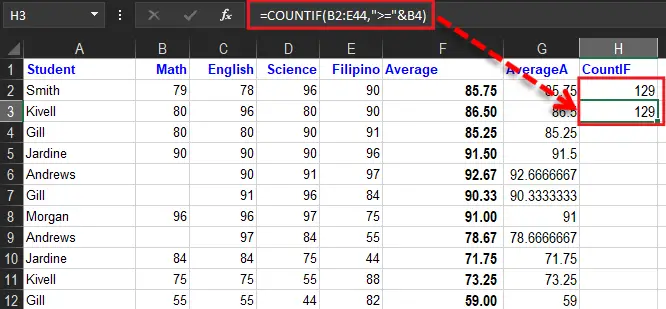# How To Use Excel Countif Function With Examples

Today we will know the power of COUNTIF function in Excel as it counts cells based on the criterion. So this article will contain examples, figures, steps and descriptions to better understand.

Prior to this function which is a function called Count in Excel. Hence, the plural counterpart of COUNTIF is COUNTIFS which is discussed in another article How To Use The COUNTIFS Function simply.

## What is Countif Function in Excel

The COUNTIF function is widely and commonly used function to count cells that meet specified criteria of a condition. Particularly, it counts cells containing dates, numbers, and even text. Notably, the COUNTIF function only caters single condition; otherwise use the COUNTIFS condition for multiple conditions or criteria.

### Syntax

`=COUNTIF(range, criteria)`

### Arguments

• `range `– The range of cells to count.
• `criteria `– The criteria that determine which cells should be counted.

### Return Value

A counted numeric value returns the function.

## Criteria

Excel function also handles criteria specifically the COUNTIF function. It supports logical operators (>,<,<>,<=,>=) and wildcards (*,?), therefore it is very useable for partial matching. The cons of this function are the syntax used to utilize the criteria.

Additionally, the countif function belongs to the basic formulas where it splits the logical criteria into two parts which are the range and criteria.

As a result, because of this design, each condition requires a separate range and criteria argument, and operators in the criteria must be enclosed in double quotes (“”).

The table below shows examples of the syntax needed for common criteria:

As you can see the last examples uses ampersand (&) character for concatenation. This explains that you will need to concatenate if you’re using another cell value, the result of the formula in criteria where uses logical operator “<“.

This is because Excel needs to evaluate cell references and formulas first to get a value before that value can be joined to an operator.

## Example

Below is the example formula you can familiarize:

• COUNTIF is not case-sensitive and that “smi” and “SMI” are treated the same.

## COUNTIF Function in Excel with Multiple Criteria Date Range

The countif function in excel also allows date as a criterion and the easiest way is to direct it into number expression cell reference or cell reference. For instance, counting cells A2:A44 contains a date greater than the date of cell B2. Hence the suggested formula is as follows:

``=COUNTIF(A2:A44, ">"&B2)``

As you can see, the date in B2 needs to concatenate operators. If you would like to use more advanced date criteria, you will need to switch on Countifs function.

Hence the safest way to utilize date as COUNTIF criterion is to use DATE function. As a result, Excel will understand the date.

For example:

Count cells in A1:A44 that contains a date more than November 11, 2022. The formula is as follows:

``=COUNTIF(A1:A44,">"&DATE(2022,11,11)) // dates less than 11-Nov-202`2`

## COUNTIF Function in Excel Greater Than

This time will know how to utilize what if in counting cells that have greater than value or greater than or equal to a value.

• The COUNTIF function below counts the number of cells that are equal to 80.
•• The COUNTIF function below counts the number of cells that are greater than or equal to 80.
•• The following COUNTIF function gives the exact same result.
•Remember: The ampersand character is used to join – greater than or equal to – and the value on the range of the cell.

## Summary

In summary, we have discussed the function of COUNTIF in Excel. Taking deeper with their examples we obtain the following:

• In matching literal question marks and asterisks type a tilde (~) in front of question mark or asterisk (i.e. ~?, ~*).
• COUNTIF formula needs a range, do not substitute with an array.
• Text strings in criteria must be enclosed in double quotes (“”).
• Cell references in criteria are not enclosed in quotes.
• The wildcard characters ? and * can be used in criteria.
• COUNTIF returns incorrect results when used to match strings longer than 255 characters.
• COUNTIF will return a #VALUE error when referencing another workbook that is closed.